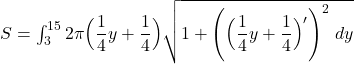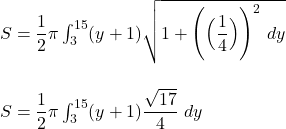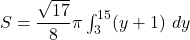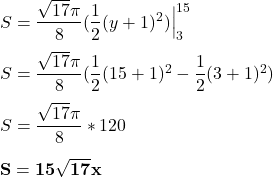## Find the area of the surface generated when the given curve is revolved about the y-axis. The part of the curve y=4x-1 between the points (1

Question

Find the area of the surface generated when the given curve is revolved about the y-axis. The part of the curve y=4x-1 between the points (1, 3) and (4, 15)

in progress 0
2 months 2021-07-25T02:27:33+00:00 1 Answers 1 views 0

Step-by-step explanation:

Let take a look at the given function y = 4x – 1 whose point is located between (1,3) and (4,15) on the graph.

Here, the function of y is non-negative. Now, expressing y in terms of x in y = 4x- 1

4x = y + 1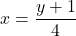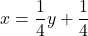By integration, the required surface area in the revolve is: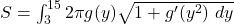where;

g(y) =